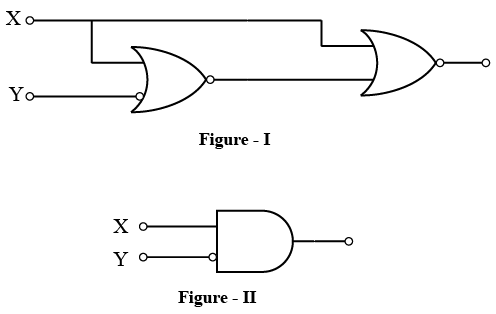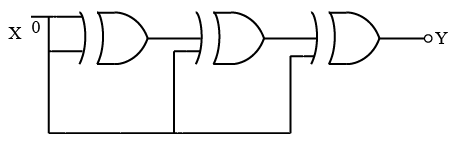# ISRO Scientist or Engineer Computer Science 2008

Instructions

For the following questions answer them individually

Question 21

# The advantage of MOS devices over bipolar devicesis thatQuestion 22

# How many 2-input multiplexers are required to construct a $$2^{10}$$ - input multiplexer ?Question 23

# A computer uses 8 digit mantissa and 2 digit exponent.If a = 0.052 and b = 28E + 11, the b + a-b willQuestion 24

# The Boolean expression $$(A + \overline{C}) (\overline{B} + \overline{C})$$ simplifies toQuestion 25

# In the expression $$\overline{A}(\overline{A} + \overline{B})$$ by writing the first term A as A + 0, the expression is best simplified aQuestion 26

# The logic operations of two combinational circuits given in Figure-I and Figure-II areQuestion 27

# The output Y of the given circuit isQuestion 28

# Whichofthe followingis not a valid rule for XOR?Question 29

# The numberof distinct simple graphs with up to three nodesisQuestion 30

# Maximum numberof edgesin a n-node undirected graph without self loops isOR